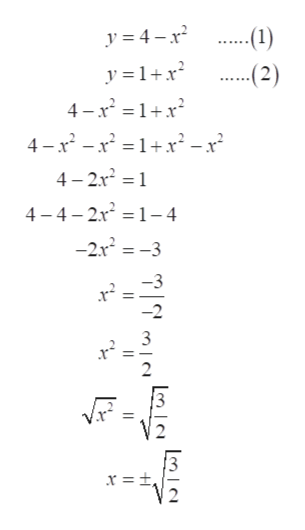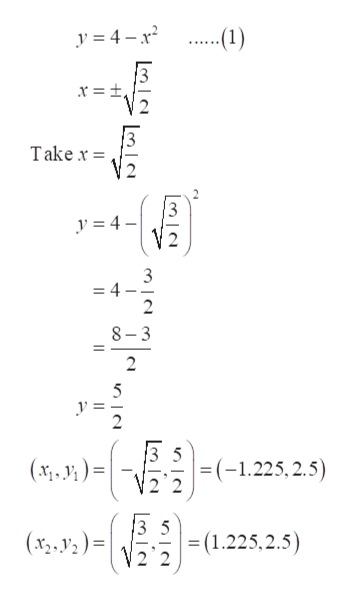# Let R be the region in the xy-plane bounded between y= 4−x2and y= 1+x2 . Set up an integral that is equal to the volume of the solid obtained by revolving R about the line x =−5. Do not evaluate the integral.

Question
81 views
Let R be the region in the xy-plane bounded between y= 4−x2
and y= 1+x2 . Set up an integral that is equal to the volume of the solid obtained by revolving R about the line x =−5. Do not evaluate the integral.
check_circle

Step 1

First of all, consider the given equations.

Step 2

Now, find the intersection points of plots 1 and 2.

Solve the equation 1 and 2 by substitution method to get the x coordinates.help_outlineImage Transcriptionclose-(1) y = 4 – x? y = 1+x? -(2) 4-x' = 1+x 4 - x - x =1+r² – x² 4 – 2.x? = 1 %3| 4 - 4- 2r = 1- 4 -2.r = -3 3 x = ±, || fullscreen
Step 3

Then, put the value of x coordinate in equation ...help_outlineImage Transcriptionclosey = 4 – x? .(1) ...... x = ±, Take x = y = 4 – 3 = 4 8– 3 3 5 =(-1.225, 2.5) (x; . V; ) = 3 5 =(1.225,2.5) 2 2 (x3, V ) = | %3D fullscreen

### Want to see the full answer?

See Solution

#### Want to see this answer and more?

Solutions are written by subject experts who are available 24/7. Questions are typically answered within 1 hour.*

See Solution
*Response times may vary by subject and question.
Tagged in

### Integration